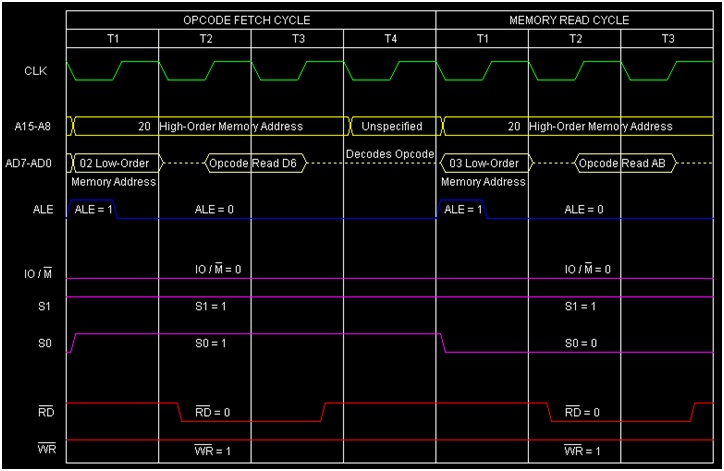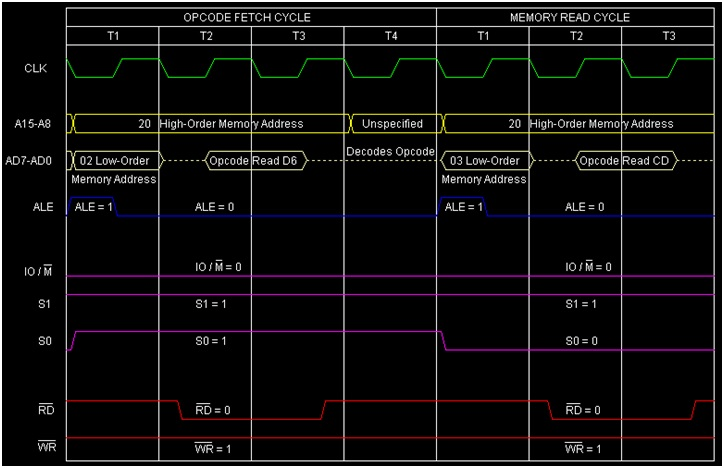# Instruction type SUI d8 in 8085 Microprocessor

In 8085 Instruction set, SUI is a mnemonic that stands for ‘SUbtract Immediate from Accumulator and here d8 stands for any 8-bit or 1-Byte data. This instruction is used to subtract 8-bit immediate data from the Accumulator. The result of the subtraction will be stored in the Accumulator over witting its previous content. As it is an arithmetic instruction, so flag bits are affected based on the result. It is a 2-Byte instruction and occupies 2-Bytes in memory.

Mnemonics, Operand Opcode(in HEX) Bytes
SUI Data D6 2

When we issue SUI d8 instruction then actually with the Accumulator’s content 2’s complement of d8 would get added. And the result will be stored back on theAccumulator.

## Example 1

SUI ABH is an example instruction of this type. It means from the Accumulator content, 2’s complement of ABH will get deducted. Let us consider the initial content of the Accumulator is CDH. Here is the tracing table.

Before After

(A)

CDH 22H

(F)

Any values Cy=0,AC=1,S=0,P=1,Z=0

2002 D6 SUI ABH Accumulator = Accumulator + 2’s Complement of ABH
2003 AB Operand ABH

Here Cy=0 indicates that result is positive. Actually A – ABH = A + 2’ Complement of ABH. Here is the calculation.

   (A)	CDH (1100 1101) ---------------> 1100 1101
2'S Complement
(d8) ABH (1010 1011) ---------------> 0101 0101
--------------
0010 0010 (22H)


Here is the timing diagram of the instruction SUI ABH instructionSummary − So this instruction SUI d8 requires 2-Bytes, 2-Machine Cycles (Opcode Fetch, Memory Read) and 7 T-States for execution as shown in the timing diagram.

## Example 1

SUI ABH is an example instruction of this type. It means from the Accumulator content, 2’s complement of ABH will get deducted. Let us consider the initial content of the Accumulator is CDH. Here is the tracing table

Before After

(A)

CDH 22H

(F)

Any values Cy=0,AC=1,S=0,P=1,Z=0

2002 D6 SUI ABH Accumulator = Accumulator + 2’s Complement of ABH
2003 AB Operand ABH

Here Cy=0 indicates that result is positive. Actually A – ABH = A + 2’ Complement of ABH. Here is the calculation.

   (A)	CDH (1100 1101) ---------------> 1100 1101
2'S Complement
(d8) ABH (1010 1011) ---------------> 0101 0101
-----------------
0010 0010 (22H)


Here is the timing diagram of the instruction SUI ABH instructionSummary − So this instruction SUI d8 requires 2-Bytes, 2-Machine Cycles (Opcode Fetch, Memory Read) and 7 T-States for execution as shown in the timing diagram.

## Example 2

SUI CDH is an example instruction of this type. It means from the Accumulator content, 2’s complement of CDH will get deducted. Let us consider the initial content of the Accumulator is ABH. Here is the tracing table.

Before After

(A)

ABH DEH

(F)

Any values Cy=1,AC=0,S=1,P=1,Z=0

2002 D6 SUI CDH Accumulator = Accumulator + 2’s Complement of CDH
2003 CD Operand CDH

Here Cy=1 indicates that result is negative. Actually A – CDH = A + 2’ Complement of CDH. Here is the calculation.

   (A)	ABH (1010 1011) ---------------> 1010 1011
2'S Complement
(d8) CDH (1100 1101) ---------------> 0011 0011
------------------
1101 1110 (DEH)


Here is the timing diagram of the instruction SUI CDH instructionSummary − So this instruction SUI d8 requires 2-Bytes, 2-Machine Cycles (Opcode Fetch, Memory Read) and 7 T-States for execution as shown in the timing diagram.